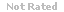Loading... Please wait...

(TCOs 3, 4) Which of the following is not true about bond indexes? (Answer)

Price:
\$18.99

Product Description

1.(TCOs 3, 4) Which of the following is not true about bond indexes?

2. (TCO 2) What does the reward to variability ratio refer to?

3. (TCO 2) The degree of risk of a particular investment is a function of ______.

4. (TCO 2) Which of the following sets of terms mean about the same thing?

5. (TCO 2) Which of the following can be said about average bond versus stock returns over the long run?

6. (TCO 2) An investor's investment opportunity set _______.

7. (TCO 2) The degree of risk of a particular investment is a function of ______.

8. (TCO 8) A disadvantage of just-in-time inventory techniques is ______.

9. (TCO 1) In the purchase and sale of securities, the "spread" refers to the

10. (TCO 1) Serial bonds _______.

11. (TCO 1) What does the derivative market refer to?

12. (TCO 1) Preferred stock has similarities to both common stock and bonds. How is it similar to bonds?

13. (TCO 1) A sinking fund bond would be of particular interest to ______.

14. (TCO 5) Which is true about callable bonds?

15. (TCO 5) The statement of cash flows _______.

16. (TCO 5) To what does the term structure of interest rates refer?

17. (TCO 5) Which of the following is true about preferred stock?

18. (TCO 5) The balance sheet ________.

19. (TCO 5) Fiscal policy ________.

20. (TCO 5) A bond's yield is determined from what?

Customers Who Viewed This Product Also Viewed

• \$18.99
• \$22.99
• \$18.99
• \$18.99
• \$8.99

• \$18.99